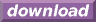#Calcute: freeware calculator

## Hyperbolic Cosecant (csch)

Calcute implements the hyperbolic cosecant function with the csch function.

```csch(1)
0.850918128239
```

It is defined as the inverse of the hyperbolic sine function csch(x) = 1 / sinh(x) or equivalently csch(x) = 2) / (exp(x) - exp(-x)). Note that this definition refers to a multiplicative inverse; the hyperbolic cosecant is not the same as the inverse hyperbolic sine (asinh) function.